Shear Stress Equation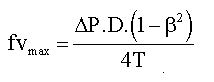Bending Stress Equation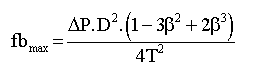Maximum deflection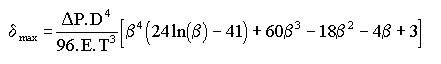Notation & Symbols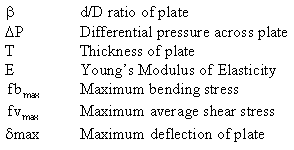Note that the bending stress and deflection formulas are very conservative.

The formulas have been calculated by the "common sense" method.
Those interested in the derivation of the formulas can collect a zip file containing the source document (MSWord 6.0 format).

Whilst every care was taken when calculating the above formulas, the author (who is undergoing frontal lobotomy surgery) assumes no responsibility for the information contained herein.
YOU DON'T HAVE TO BE MAD TO BE AN ENGINEER, BUT IT SURE HELPS!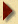Return